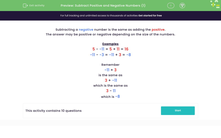# Subtract Positive and Negative Numbers (1)

In this worksheet, students subtract positive and negative numbers.Key stage:  KS 3

Curriculum topic:   Number

Curriculum subtopic:   Use Four Operations for All Numbers

Difficulty level:#### Worksheet Overview

Subtracting a negative number is the same as adding the positive.

The answer may be positive or negative depending on the size of the numbers.

Examples

5 - -11 = 5 + 11 = 16

-11 - -3 = -11 + 3 = -8

Remember

-11 + 3

is the same as

3 + -11

which is the same as

3 - 11

which is -8

### What is EdPlace?

We're your National Curriculum aligned online education content provider helping each child succeed in English, maths and science from year 1 to GCSE. With an EdPlace account you’ll be able to track and measure progress, helping each child achieve their best. We build confidence and attainment by personalising each child’s learning at a level that suits them.

Get started